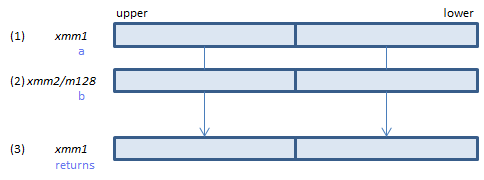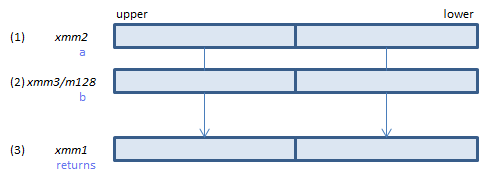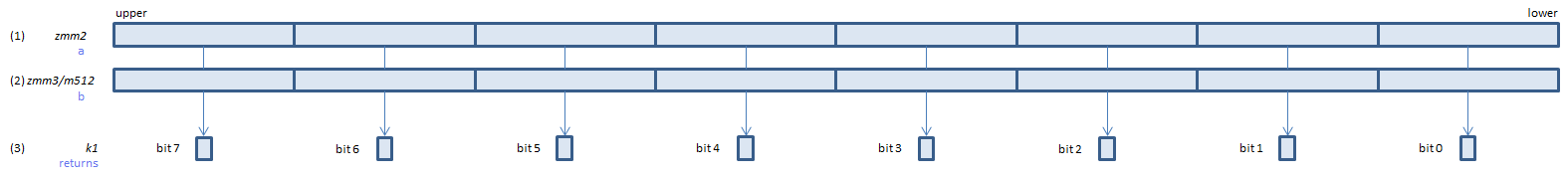﻿ cmpnltpd

## CMPNLTPD - CoMPare Not Less Than Packed Double

CMPNLTPD xmm1, xmm2/m128    (S2
__m128d _mm_cmpnlt_pd(__m128d a, __m128d b)For each double, if (1) >= (2) or either or both of (1)(2) is NaN, set 1, else set 0, to all bits of the corresponding double of (3).
VCMPNLTPD xmm1, xmm2, xmm3/m128    (V1
__m128d _mm_cmpnlt_pd(__m128d a, __m128d b)For each double, if (1) >= (2) or either or both of (1)(2) is NaN, set 1, else set 0, to all bits of the corresponding double of (3).
VCMPNLTPD ymm1, ymm2, ymm3/m256    (V1
__m256d _mm256_cmp_pd(__m256d a, __m256d b, _CMP_NLT_US)For each double, if (1) >= (2) or either or both of (1)(2) is NaN, set 1, else set 0, to all bits of the corresponding double of (3).
VCMPNLTPD k1{k2}, xmm2, xmm3/m128/m64bcst    (V5+VLFor each double, if (1) >= (2) or either or both of (1)(2) is NaN, set 1, else set 0, to the corresponding bit of (3).
If k2 bit is 0, the comparison is not done and the corresponding bit of (3) is set to zero. Upper bits of (3) are zero cleared.
VCMPNLTPD k1{k2}, ymm2, ymm3/m256/m64bcst    (V5+VLFor each double, if (1) >= (2) or either or both of (1)(2) is NaN, set 1, else set 0, to the corresponding bit of (3).
If k2 bit is 0, the comparison is not done and the corresponding bit of (3) is set to zero. Upper bits of (3) are zero cleared.
VCMPNLTPD k1{k2}, zmm2, zmm3/m512/m64bcst{sae}    (V5